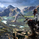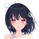# HEMA - A Fast And Efficient Estimate Of The Hull Moving Average

2061 görüntülenme
Introduction

The Hull moving average ( HMA ) developed by Alan Hull is one of the many moving averages that aim to reduce lag while providing effective smoothing. The HMA make use of 3 linearly weighted ( WMA ) moving averages, with respective periods p/2, p and √p, this involve three convolutions, which affect computation time, a more efficient version exist under the name of exponential Hull moving average (EHMA), this version make use of exponential moving averages instead of linearly weighted ones, which dramatically decrease the computation time, however the difference with the original version is clearly noticeable.

In this post an efficient and simple estimate is proposed, the estimation process will be fully described and some comparison with the original HMA will be presented.

This post and indicator is dedicated to LucF

Estimation Process

Estimating a moving average is easier when we look at its weights (represented by the impulse response), we basically want to find a similar set of weights via more efficient calculations, the estimation process is therefore based on fully understanding the weighting architecture of the moving average we want to estimate.

The impulse response of an HMA of period 20 is as follows :

We can see that the first weights increases a bit before decaying, the weights then decay, cross under 0 and increase again. More recent closing price values benefits of the highest weights, while the oldest values have negatives ones, negative weighting is what allow to drastically reduce the lag of the HMA . Based on this information we know that our estimate will be a linear combination of two moving averages with unknown coefficients :

a × MA1 + b × MA2

With a > 0 and b < 0, the lag of MA1 is lower than the lag of MA2. We first need to capture the general envelope of the weights, which has an overall non-linearly decaying shape, therefore the use of an exponential moving average might seem appropriate.

In orange the impulse response of an exponential moving average of period p/2, that is 10. We can see that such impulse response is not a bad estimate of the overall shape of the HMA impulse response, based on this information we might perform our linear combination with a simple moving average :

2EMA(p/2) + -1SMA(p)

this gives the following impulse response :

As we can see there is a clear lack of accuracy, but because the impulse response of a simple moving is a constant we can't have the short increasing weights of the HMA , we therefore need a non-constant impulse response for our linear combination, a WMA might be appropriate. Therefore we will use :

2WMA(p/2) + -1EMA(p/2)

Note that the lag a WMA is inferior to the lag of an EMA of same period, this is why the period of the WMA is p/2. We obtain :

The shape has improved, but the fit is poor, which mean we should change our coefficients, more precisely increasing the coefficient of the WMA (thus decreasing the one of the EMA ). We will try :

3WMA(p/2) + -2EMA(p/2)

We then obtain :

This estimate seems to have a decent fit, and this linear combination is therefore used.

Comparison

HMA in blue and the estimate in fuchsia with both period 50, the difference can be noted, however the estimate is relatively accurate.

In the image above the period has been set to 200.

Conclusion

In this post an efficient estimate of the HMA has been proposed, we have seen that the HMA can be estimated via the linear combinations of a WMA and an EMA of each period p/2, this isn't important for the EMA who is based on recursion but is however a big deal for the WMA who use recursion, and therefore p indicate the number of data points to be used in the convolution, knowing that we use only convolution and that this convolution use twice less data points then one of the WMA used in the HMA is a pretty great thing.

Subtle tweaking of the coefficients/moving averages length's might help have an even more accurate estimate, the fact that the WMA make use of a period of √p is certainly the most disturbing aspect when it comes to estimating the HMA . I also described more in depth the process of estimating a moving average.

I hope you learned something in this post, it took me quite a lot of time to prepare, maybe 2 hours, some pinescripters pass an enormous amount of time providing content and helping the community, one of them being LucF, without him i don't think you'll be seeing this indicator as well as many ones i previously posted, I encourage you to thank him and check his work for Pinecoders as well as following him.

« Je suis las des cruautés de mes semblables, qui ne sont pas mes pareils.

« Je prendrai l’essor et je m’envolerai vers la mer.

« Je connaîtrai le goût des brises du large. J’entendrai les grands cris de la tempête.

## YorumlarStraight and 2 the Point. Love the Analysis and Comparison Charts.
Cevap GönderMnbrass549